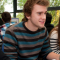# Velocity / Acceleration / Time Calculator

Velocity / Acceleration / Time Calculator

•Baker says:

A bicycle travels 3.2 km due east in 0.10 h, then 4.8 km at 15◦
east of north in 0.15 h,
and finally another 3.2 km due east in 0.10 h to reach its destination. The time lost in
turning is negligible. What was the average velocity for the entire trip?

•Shenal Priyashna says:

An object experiences a displacement of 1.13m towards the right. If the object’s final position is 25cm right of the origin,then what is the objects original position?

•Riya says:

A horizontal shelf moves vertically with simple harmonic motion of period 1.5 sec. What is the greatest amplitude that the shelf can have so that the objects resting on it never leav it?

•ben says:

A 20 kg object moves at a constant speed of 10 m/s due north in 10 s. What is the acceleration of the object ?

•Ammaarah Mahomed says:

When speed or velocity is constant acceleration is 0

•ben says:

What is the potential gravitational energy of a 90 kg ski-jumper at the top of 80m hill ?

•Peter Druker says:

Gravitational potential energy
Kinetic energy = 692 kj (kilojoules)
192.2 Wh (watt hours)
0.1922 kWh (kilowatt hours)
Calculator here http://geekandnerd.org/gravitational-potential-energy-calculator/

•ben says:

A river has current with a velocity of 1.0 m/s south. A boat whose speed in still water is 5.0 m/s is directed east across the 100 m wide rive.How far downstream will the boat travel and the relative velocity of the boat will move to the shore.

•Ammaarah Mahomed says:

A car is travelling at a uniform speed of 30m/s maintaining a distance of 35m behind a truck travelling atthe same uniform speed.
The truck notices an obstacle and accelerates uniformly by pressing the brakes and coming to a stop which takes 12s
1. calc the acceleration of the truck slowing down
2. How far does the truck travel when slowing down during the 12s
3. If the car continues in its original motion hiw far ahead will it stop of the truck
It was in my paper and I wanna know if I did it ryt

•Festus Sando says:

tina threw a ball to a height of a flagpole 25 meter high. how long will it take the ball to reach that height and dropped back on the ground if the ball accelerates at 0.5m/s2

•Ali says:

aunter stands at the eadgof acliff 85.0m whenhe seesawild boar directly belowhim.needing to feed his village ,he ime his arrow 60 above the horizontal and releases his arrow. at theexact same instant the wild boar knowwing she has being hunted runs away horzontly from the hunter at aconstant speed of 12.5m/s. find where relative his initial position and find the velocity of the arrow as it strikes the boar

•Tonywise says:

A salon car started at 5m/s,then ,accelerates uniformly for 4s. Then, it attained full velocity and move uniformly for 6s. The car was brought to rest with uniform retardation of 1.375 m/s2 for 8s. (Draw a velocity – time graph). calculate the distance covered.

•Ramakanta lenka says:

A stone is thrown vertically upward with an initial velocity v from top of a top of a tower reaches the ground with velocity 3m.The height of tower

•Morgan says:

Bob (68 kg) goes down the water slide shown above. The ride begins at a height of 37 meters. The smaller hill is only 16 meters tall. At the end of the ride Bob is stopped by a giant rubber band stretched across the bottom of the slide. (Use your imagination.)

How fast will Bob be moving at the top of the small hill? (Ignore friction.)

•Fatima says:

If the position of a partical is given by x= 20 t3 -5 t3 where x is in meters and t is in seconds if ever is the particals velocity 0 ? when is its acceleration 0 ?

•Yassen says:

a stone of mass 600 gm attached to a string of length 10 cm, rotating at velocity of 3 m/s calculate the centripetal force and centripetal acceleration

•Leekay jimenez says:

A dolphin decelerates for 2.0 sec feom an initial speed of 7.5 m/sec in order to take a look at a dolphin of the opposite sex. Calculate the dolphin’s final speed if the deceleration had a magnitude of 1.5m/sec^2

•david says:

A 3kg hammer is used to drive a nail into a piece of wood.if at the time of impact the speed of the hammer is 5m/s and it drive the nail 1cm to the wood,calculate
i)The acceleration
ii)the force exerted

•vikas latil says:

A body starts with some initial velocity along a straight line. After 3 second it is found to be at a distance of 15 metre from the starting point. At 4 second it is at starting point. What is the initial velocity of the body? ( Assume constant acceleration)

•Moin says:

Can velocity and acceleration be in different directions.give two reasons

•RND says:

If France has two cars that accelerates at a rate of 80 meter per second squared and car A has a mass of 1000 kg while car B has a mass of 2000 kg. How much force is needed by the two cars to have the acceleration? Which of them needs more force to accelerate?

•chandan says:

A train 100m long travelling at 40m\s starts overtaking another train 200m long travelling at 30m\s.What is the time taken by the first traint pass the second train completely?

•lo says:

What is the acceleration due to gravity of a planet with mass of 6.54 * 10^24 kg and radius of 5.73 * 10^6 m?